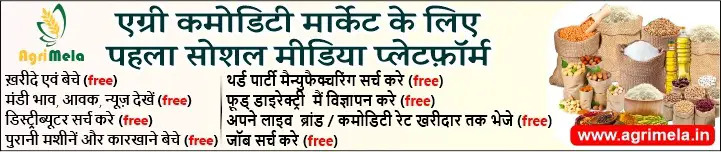Rate this

Rate this

Rate this

Rate this

Rate this

Rate this

Rate this

Rate this

Rate this

Rate this

Rate this

## Nakoda Canvassers

Rate this

Edible Oil Seed Broker, Food Grain Broker, Pulses Broker, Rice Broker in Nalkhera, Madhya Pradesh, India

Rate this

Rate this

Rate this

Rate this

Rate this

Rate this

Rate this

Rate this

Rate this

Rate this

Rate this

Rate this

Rate this